### IMO Shortlist 2013 problem G4

Kvaliteta:
Avg: 3,0
Težina:
Avg: 7,0
Let$ABC$ be a triangle with$\angle B > \angle C$. Let$P$ and$Q$ be two different points on line$AC$ such that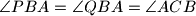$\angle PBA = \angle QBA = \angle ACB$ and$A$ is located between$P$ and$C$. Suppose that there exists and interior point$D$ of segment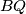$BQ$ for which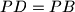$PD = PB$. Let the ray$AD$ intersect the circle$ABC$ at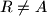$R \neq A$. Prove that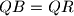$QB = QR$.
Izvor: Georgia